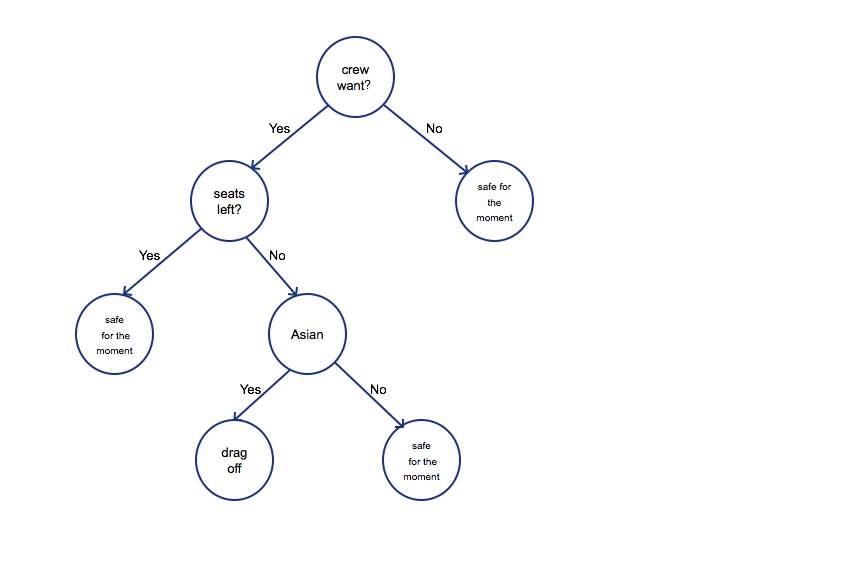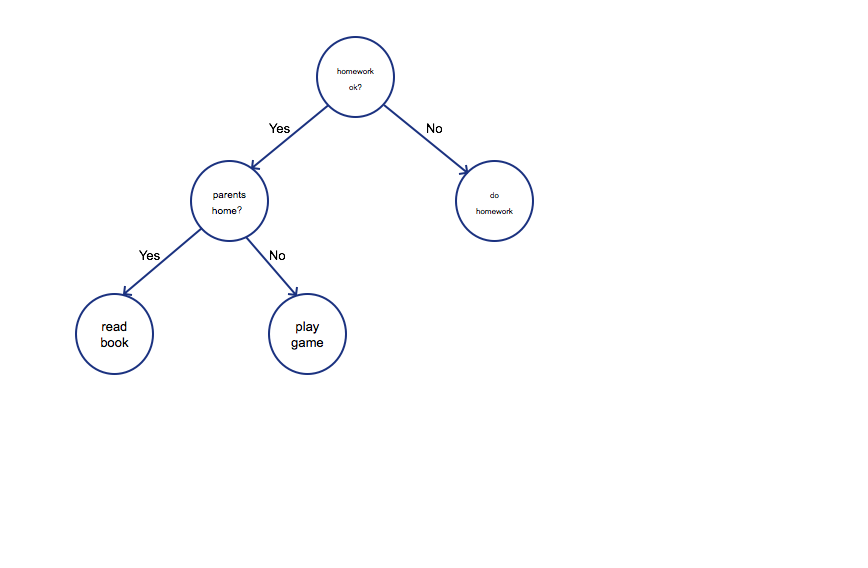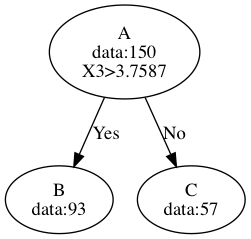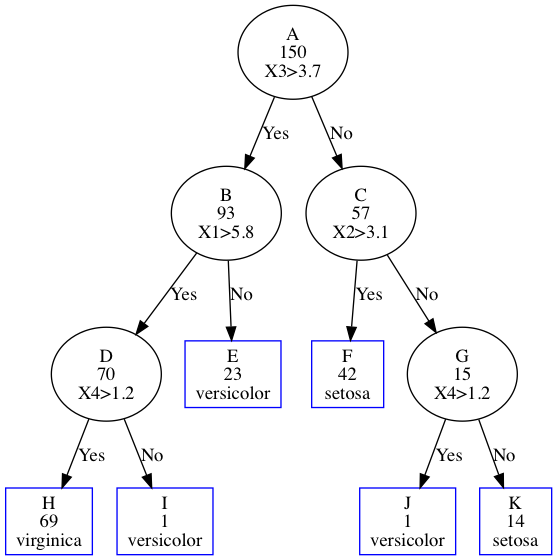## Preface

In April 9th, 2017, incident occurred in United Airlines where crew of UA beat up a passenger and dragged him out of the plane before which was about to take off attracted attention all around the world. Many would gave out doubt: why a company being so rude to passengers can exist in this world? Actually, UA is going well is just because they have an extremely precise emergency situation procedure which is calculate by compute depending on big-data analysis. Computer can help us make decisions though, it has no emotions, which is effective in most cases, but can not be approved by our human beings. Let’s take a look at how algorithm make a decision:It is a decision tree, which simply represents the procedure of how UA algorithm make the decision. First of all, before taking off, four employees of UA need fly from Chicago to Kentucky. Then the algorithm check if there is any seats left, if so, passengers were safe for the moment. But UA3411 was full, the algorithm began assessing the importance of employees or passengers. Obviously, the algorithm think crew is more important due to business consideration. Then how to choose who should be evicted from the plane. The algorithm was more complicated than the tree I drew, however, Asian or not was one of the criterion. But why? Because Asian are pushovers. The passenger agreed at first, however, when he heard that he had to wait for one day, he realized that he could not treat his patient, then he refused. Then he was beat up and dragged off the plane.

As you have seen, it is a decision tree, which is similar to human decision-making process. Decision tree is a simple but powerful algorithm in machine learning. In fact, you are often using decision tree theory when making decision, for example## Introduction

Decision tree is a classification and regression algorithm, we build a tree through statistics. Today we only talk about how to classify dataset using Decision Tree. First we will introduce some information theory background knowledge, then we use iris data build a decision tree using IDC3 algorithm.

## Iris data

Iris dataset is a very famous dataset deposited on UCI machine learning repository, which described three kinds of iris. there are four columns corresponding for features as followed：

• sepal length in cm
• sepal width in cm
• petal length in cm
• petal width in cm

The last column represents iris categories:

• Iris-setosa (n=50)
• Iris-versicolor (n=50)
• Iris-virginica (n=50)

Here, our task is to use the dataset to train a model and generate a decision tree. During the process we need calculate some statistics values to decide how to generate a better one.

The dataset is very small so that you can easily download it and take a look.

## Entropy and Information Gain

#### Entropy

Before Decision Tree, I’d like to talk about some concept in Information Theory. Entropy is a concept from thermodynamics at first, C.E.Shannon introduced which into information theory which represent redundancy in 1948. It sounds a very strange concept. In fact, it is very easy to understand. For example, during the knockout stages in world Cup Games, there are 16 teams. Now I let you guess which team will win the champion which assume I know the answer, how many times do you need to get the outcome? First of all, you cut 16 teams to 8-8 parts, you asked me if the team in first 8 teams or the other. I told you that the team was in the other 8 teams. Then you cut the the 8 teams again, you ask me if the team is in the first 4 teams or the other, I told you that the champion would be in the first 4 teams, and so forth and so on. And how many times is the entropy of who wining the champion.

$$Entropy(champion) = {\rm log}_2^{16}=4$$

That is, we can use 4 bits to represents which team will win the game. Clever you may ask why we divide team to two parts other than three or four parts. That is because we use binary represents the world in computer world. $2^4=16$ means we can use 4 bit represents 16 conditions. We can use entropy represent all information in this world. And if you have known that which team will win the campion, the entropy is 0, because, you do not need any more information to deduce the outcome.

Entropy represents uncertainty indeed. Ancient China, we have to record history on bamboo slips, which demanded us decrease words. That means entropy of every single ancient Chinese character is higher than words we are saying today. That is, if we lost just some of these words, we would lose lots of stories. There are many songs starts with:”Yoo, yoo, check now”, which barely offer us information, which means we can drop those words and interpret the these songs precisely as well. The entropy of these sentence is low.

Assume $X$ is discrete random variable, the distribution is:
$$P(X=x_i)=p_i$$
then the entropy of X is:
$$H(X)=-\sum_{i=1}^{n}p_i {\rm log}_2 p_i$$
where if p_0=0, we define 0log0 = 0.

It seems that the equation has nothing to do with the entropy we have calculated in the champion example. Now let’s calculate the example. First of all $X$ represents the probability of each team which would win the game. we assume all teams were at the same level, so we have
$$p(X=x_1)=p(X=x_2)=p(X=x_3)=\cdots = p(X=x_{16})=\frac{1}{16}$$
the entropy is
$$H(X)=-\sum_{i=1}^{16}\frac{1}{16}{\rm log}_2 \frac{1}{16}=-16\times\frac{1}{16}\times {\rm log}_2 {2^{-4}}=4$$

Bingo, the the answer is same. In fact, if we know some more information, the entropy is lower than 4. for example, the probability of Germany is higher than some Asian teams.

#### Entropy and Iris Data

Now we calculate entropy of Iris Data which will be used to fit a decision tree in following sections. We concern about the categories(setosa, versicolor and virginica). Remember the equation of how to calculate entropy:
$$H(X)=-\sum_{i=1}^{n}p_i {\rm log}_2 p_i$$

Three kinds of flowers are all 50s, so the probability of each category is the same:
$$p_1=p_2=p_3=\frac{50}{50+50+50}=\frac{1}{3}$$
Then, the entropy is pretty easy to calculate
$$H(X)=-1\times (\frac{1}{3}{\rm log}_2\frac{1}{3}+\frac{1}{3}{\rm log}_2\frac{1}{3}+\frac{1}{3}{\rm log}_2\frac{1}{3})=1.5850$$

#### Conditional Entropy

The meaning of Conditional Entropy is as its name. With respect with random variable$(X, Y)$, the joint distribution is
$$P(X=x_i, Y=y_j)=p_{ij}, i=1,2,3\cdots m; j=1,2,3,\cdots n$$
Conditional Entropy H(Y|X) represents that given we have known random variable $X$ , the disorder or uncertainty of $Y$. The definition is as followed:
$$H(Y|X)=\sum_{i=1}^m p_i H(Y|X=x_i)$$
Here, $p_i=P(X=x_i)$.

#### Conditional Entropy and Iris Data

We calculate some Conditional Entropy as examples. First of all, I random choose 15 columns of sepal length with respect to their categories. the result is as followed：

No. sepal length in cm categories
1 5.90 Iris-versicolor
2 7.20 Iris-virginica
3 5.00 Iris-versicolor
4 5.00 Iris-setosa
5 5.90 Iris-versicolor
6 5.70 Iris-setosa
7 5.20 Iris-versicolor
8 5.50 Iris-versicolor
9 4.80 Iris-setosa
10 4.60 Iris-setosa
11 6.50 Iris-versicolor
12 5.20 Iris-setosa
13 7.70 Iris-virginica
14 6.40 Iris-virginica
15 6.00 Iris-versicolor

The octave code is

We just take this 15 items for examples, I assume that we divide sepal length into two parts: greater than mean and less than mean. The mean is
$$mean = (5.90+7.2+\cdots+6.00)/15 = 5.7733$$
There are 8 elements less then 5.7733 and 7 bigger ones. That is

mean idx of greater than mean idx of less than mean
5.7733 1,2,5,11,13,14,15 3,4,6,7,8,9,10,12

We let $x_1=greater$(1,2,5,11,13,14,15), $x_2=less$(3,4,6,7,8,9,10,12) then
$$H(Y|X=x_1)=-(p_1 {\rm log}_2 p_1 + p_2 {\rm log}_2 p_2 + p_3 {\rm log}_2 p_3)=\frac{4}{7}{\rm log}_2\frac{4}{7}+\frac{3}{7}{\rm log}_2\frac{3}{7}+0{\rm log}_2 0=0.98523$$
$$H(Y|X=x_2)=-(p_1 {\rm log}_2 p_1 + p_2 {\rm log}_2 p_2+p_3 {\rm log}_2 p_3)=\frac{3}{8}{\rm log}_2\frac{3}{8}+0{\rm log}_2 0+\frac{5}{8}{\rm log}_2\frac{5}{8}=0.95443$$

The Conditional Entropy then is
$$H(Y|X)=\sum_{i=1}^{2}p_i H(Y|x_i)=\frac{7}{15}\times 0.98523+\frac{8}{15}\times 0.95443=0.96880$$

#### Information Gain

Just as its name implies, Information Gain means the information we have gained after adding some features. That is, we can vanish some uncertainty when we add some information. For example, I want you to guess an NBA player, the uncertainty is very high, however, there are only several persons in the list if I tell you that he is a Chinese. You gained information after knowing the Chinese feature to decrease the uncertainty. The calculation of Information Gain is
$$IG(Y, X)= H(Y)-H(Y|X)$$
Here, we want to decide $Y$ with feature $X$. It is easy, just Entropy of $Y$ minus Conditional Entropy $Y$ given $X$. The meaning is obvious too: $H(Y)$ represents uncertainty, $H(Y|X)$ represents uncertainty of $Y$ given $X$, the difference is the Information Gain.

#### Information Gain and Iris Data

In this section, I will apply Information Gain equations to the whole Iris data. First of all, let $Y$ represent categories of iris, and $X_1,X_2,X_3, X_4$ represent sepal length, sepal width, petal length petal width respectively.

We have computed that $H(Y)=1.0986$, next, we will calculate 4 Conditional Entropy $H(Y|X_1),H(Y|X_2),H(Y|X_3),H(Y|X_4)$. In light of continuousness of $X$, we divide them by mean of each feature. Then
$$\overline{X_1}=5.8433,\,\overline{X_2}=3.0540,\,\overline{X_3}=3.7587,\,\overline{X_4}=1.1987$$

$$H(Y|X_1)=-\sum_{i=1}^3 p_i H(Y|X_{1i})=-(\frac{70}{150}(\frac{0}{70}{\rm log}_2\frac{0}{70}+\frac{26}{70}{\rm log}_2\frac{26}{70} +\frac{44}{70}{\rm log}_2\frac{44}{70})+\frac{80}{150}(\frac{50}{80}{\rm log}_2\frac{50}{80}+\frac{24}{80}{\rm log}_2\frac{24}{80}+\frac{6}{80}{\rm log}_2\frac{6}{80}))=1.09757$$

$$H(Y|X_2)=-\sum_{i=1}^3 p_i H(Y|X_{2i})=-(\frac{67}{150}(\frac{42}{67}{\rm log}_2\frac{42}{67}+\frac{8}{67}{\rm log}_2\frac{8}{67}+\frac{17}{67}{\rm log}_2\frac{17}{67}+\frac{83}{150}(\frac{8}{83}{\rm log}_2\frac{8}{83}+\frac{42}{83}{\rm log}_2\frac{42}{83}+\frac{33}{83}{\rm log}_2\frac{33}{83}))=1.32433$$

$$H(Y|X_3)=-\sum_{i=1}^3 p_i H(Y|X_{3i})=-(\frac{93}{150}(\frac{0}{93}{\rm log}_2\frac{0}{93}+\frac{43}{93}{\rm log}_2\frac{43}{93}+\frac{50}{93}{\rm log}_2\frac{50}{93}+\frac{57}{150}(\frac{50}{57}{\rm log}_2\frac{50}{57}+\frac{7}{57}{\rm log}_2\frac{7}{57}+\frac{0}{57}{\rm log}_2\frac{0}{57}))=0.821667$$

$$H(Y|X_4)=-\sum_{i=1}^3 p_i H(Y|X_{4i})=-(\frac{90}{150}(\frac{0}{90}{\rm log}_2\frac{0}{90}+\frac{40}{90}{\rm log}_2\frac{40}{90}+\frac{50}{90}{\rm log}_2\frac{50}{90}+\frac{60}{150}(\frac{50}{60}{\rm log}_2\frac{50}{60}+\frac{10}{60}{\rm log}_2\frac{10}{60}+\frac{0}{60}{\rm log}_2\frac{0}{60}))=0.854655$$
Information Gains is easy to get
$$IG(Y, X_1)=H(Y)-H(Y|X_1)=1.5850-1.09757=0.487427$$

$$IG(Y, X_2)=H(Y)-H(Y|X_2)=1.5850-1.32433=0.260669$$

$$IG(Y, X_3)=H(Y)-H(Y|X_3)=1.5850-0.821667=0.763333$$

$$IG(Y, X_4)=H(Y)-H(Y|X_4)=1.5850-0.854655=0.730345$$
By now, we find that $IG(Y, X_3)$ is bigger than others, which means feature $X_3$ supplies more information.

## ID3(Iterative Dichotomiser 3)

ID3 algorithm was developed by Ross Quinlan in 1986, which is a very classic algorithm as well as C4.5 and CART. We First apply Information Gain of each feature with respect to iris data. Then to choose the maximum to divide data into 2 parts. For each part we apply Information Gain recursively until we put all parents data to one node. Now that we have know Information Gain from the last section, obviously we choose X3 as the feature dividing data into 2 parts in the first place.Let’s take a look at the first cut using feature $X_3$. We have 150 items at first, after comparing if $X_3>3.7587$, we divide data into two parts, one has 93 items, the other got 57. From the data, we know that there is no setosa in node B, meanwhile, no virginica in node C, which means that this feature is very good for split data due to exclude setosa and virginica.

Node B Node C
setosa 0 50
versicolor 43 7
virginica 50 0

The end condition of the algorithm is decided by IG. When IG is less then some threshold or if there is only one category left, we can end the algorithm. If IG less than some value(e.g. 0.01) and more than one category left simultaneously, we have to choose a final category to be the leaf, the rule is to set the category having samples more than the others.

Take Node H for example, we set IG threshold to 0.01 in the first place. Then we calculate the Information Gain for each feature, the biggest IG from feature 2(sepal width in cm), which is 0.003204 and less than 0.01. So we have to set H as a leaf. There are 0 Iris-setosa, 25 Iris-versicolor and 44 Iris-virginica in the leaf, so we set the bigger one(i.e. Iris-virginica) to the leaf.## Summary

Today we have talked about what is decision tree algorithm. Firstly, I introduce three background concept Entropy, Conditional Entropy and Information Gain. Next we apply ID3 algorithm to Iris data to build a decision.

One of the most significant advantages of decision tree is that we can explain the result. If the algorithm decided UA should beat the their passengers, they could trace the tree to find the path of reason chain. It is very useful to tell consumers why we recommend them something, under such circumstance, we can use decision tree to train a model.

There is a shortcoming that Information Gain tends to use feature with more values. In order to resolve the problem, Ross Quinlan improved the algorithm through Information Gain Rate Rather than IG. Breiman introduced CART algorithm subsequently, which can be applied to classification as well as regression. Recently, Scientists have developed more powerful algorithm such as Random Forest and Gradient Boosting Decision Tree etc.